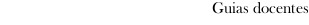Educational guide 2017_18Facultade de Química
 Grao en QuímicaSubjectsPhysics: Physics IContents
 Topic Sub-topic 1. DESCRIPTION OF THE PHYSICAL REALITY Introduction - Physical magnitudes and units - Dimensional analysis  Errors. 2. KINEMATICS OF THE POINT AND RIGID BODY Material point - Vector position, velocity and acceleration - Tangent and normal components of the acceleration - Study of some movements: rectilinear and plane - Rigid body. 3. PRINCIPLES OF THE DYNAMICS Concept of force - Newton Laws - Newton´s theory of gravitation. 4. DYNAMICS OF THE PARTICLE Equations of motion - Momentum and angular momentum - Radial Forces: Conservation of the angular momentum - Work and power - Kinetic Energy - Conservation of the mechanical energy - Non conservative forces. The conservation of energy. - Energy diagrams. 5. OSCILLATING MOTION Simple harmonic Motion: Kinematics, Dynamics and Energy. 6. DYNAMICS OF SYSTEMS OF PARTICLES Internal and external forces - Equation of motion for the center of mass - Work of external and internal forces  Collisions. 7. THE RIGID BODY Rigid Body: Rotational motion: Moment of inertia, angular momentum, Kinetic Energy. 8. FLUIDS Pressure and density. Pressure in a fluid at rest. Measurement of pressure  Surface Tension Capillarity. Jurin´s Law  Tate´s Law. 9. INTRODUCTION TO THE THERMODYNAMICS. THERMOMETRY Macroscopic and microscopic description - Thermal equilibrium - Zero´th law of Thermodynamics. Temperature  Measure of temperature. Thermometers - Ideal Gas. Ideal gas temperature scale. 10. HEAT AND WORK Thermodynamic Equilibrium. Equations of state. Quasiestatic Processes - Thermodynamic work - Heat capacity and specific heat. Latent heat. 11. THE FIRST LAW OF THERMODYNAMICS The First Law of Thermodynamics - Internal Energy, enthalpy and heat capacities of the ideal gases. Mayer´s Law -Adiabatic changes of an ideal gas. 12. THE SECOND LAW OF THERMODYNAMICS Introduction - Second Law: Clausius and Kelvin-Planck Statements - Cycle of Carnot. Theorem of Carnot- Thermodynamic Scale of Temperatures - Inequality of Clausius- Entropy.
 Universidade de Vigo            | Reitoría | Campus Universitario | C.P. 36.310 Vigo (Pontevedra) | Spain | Tlf: +34 986 812 000